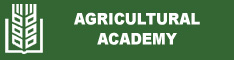The Egg Production Efficiency Index (EPEI) as an economic indicator for measuring poultry egg production
Hristo Lukanov, Atanas Genchev, Todor Petrov
Abstract: The aim of the study was to introduce an up-to-date index for evaluation of economic efficiency of egg production in domestic fowl. The proposed egg production efficiency index (EPEI) was calculated using the following equation: EPEI = [(L × DEMP)/FCR)] × 100, where: L – Liveability (%); DEMP – Daily egg mass produced (kg); FCR – Feed conversion ratio (kg/ kg egg mass). DEMP = (HDEP x AEW)/t, where HDEP – Hen-Day egg production (number); AEW – Average egg weight
(kg); t – period (days). The ideal egg production efficiency index, calculated on the basis of a full production period, equals 310. For a production period of 52-81 weeks, EPEI of 230 may be estimated as a threshold for modern white and brown egg layer hybrids. EPEI may be also applied for monitoring of efficiency of egg production dynamics in a given group of stock layer hens. The index may be also used in other domestic fowl species.
Keywords: egg production; egg weight; FCR; production efficiency; table eggs
Date published: 2023-08-21